# Chapter 24Envelopes of Surfaces in 3D

Michal Meyerovitch, Ron Wein, and Baruch Zukerman

## Table of Contents

 24.1 Introduction 24.2 The Envelope-Traits Concept 24.3 Examples 24.3.1   Example for Envelope of Triangles 24.3.2   Example for Envelope of Spheres 24.3.3   Example for Envelope of Planes

## 24.1   Introduction

A continuous surface S in3 is called xy-monotone, if every line parallel to the z-axis intersects it at a single point at most. For example, the sphere x2 + y2 + z2 = 1 is not xy-monotone as the z-axis intersects it at (0, 0, -1) and at (0, 0, 1); however, if we use the xy-plane to split it to an upper hemisphere and a lower hemisphere, these two hemispheres are xy-monotone.

An xy-monotone surface can therefore be represented as a bivariate function z = S(x,y), defined over some continuous range RS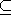2. Given a set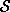= { S1, S2, ..., Sn } of xy-monotone surfaces, their lower envelope is defined as the point-wise minimum of all surfaces. Namely, the lower envelope of the setcan be defined as the following function: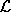(x,y) = min1knSk (x,y) ,

where we define Sk(x,y) = Sk(x,y) for (x,y)RSk, and Sk(x,y) =otherwise.

Similarly, the upper envelope ofis the point-wise maximum of the xy-monotone surfaces in the set: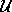(x,y) = max1knSk (x,y) ,

where in this case Sk(x,y) = -for (x,y) RSk.

Given a set of xy-monotone surfaces, the minimization diagram ofis a subdivision of the xy-plane into cells, such that the identity of the surfaces that induce the lower diagram over a specific cell of the subdivision (be it a face, an edge, or a vertex) is the same. In non-degenerate situation, a face is induced by a single surface (or by no surfaces at all, if there are no xy-monotone surfaces defined over it), an edge is induced by a single surface and corresponds to its projected boundary, or by two surfaces and corresponds to their projected intersection curve, and a vertex is induced by a single surface and corresponds to its projected boundary point, or by three surfaces and corresponds to their projected intersection point. The maximization diagram is symmetrically defined for upper envelopes. In the rest of this chapter, we refer to both these diagrams as envelope diagrams.

It is easy to see that an envelope diagram is no more than a planar arrangement (see Chapter 20), represented using an extended DCEL structure, such that every DCEL record (namely each face, halfedge and vertex) stores an additional container of it originators: the xy-monotone surfaces that induce this feature.

Lower and upper envelopes can be efficiently computed using a divide-and-conquer approach. First note that the envelope diagram for a single xy-monotone curve Sk is trivial to compute: we project the boundary of its range of definition RSk onto the xy-plane, and label the faces it induces accordingly. Given a set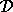of (non necessarily xy-monotone) surfaces in3, we subdivide each surface into a finite number of weakly xy-monotone surfaces,1 and obtain the set. Then, we split the set into two disjoint subsets1 and2, and we compute their envelope diagrams recursively. Finally, we merge the diagrams, and we do this by overlaying them and then applying some post-processing on the resulting diagram. The post-processing stage is non-trivial and involves the projection of intersection curves onto the xy-plane - see [Mey06] for more details.

## 24.2   The Envelope-Traits Concept

The implementation of the envelope-computation algorithm is generic and can handle arbitrary surfaces. It is parameterized with a traits class, which defines the geometry of surfaces it handles, and supports all the necessary functionality on these surfaces, and on their projections onto the xy-plane. The traits class must model the EnvelopeTraits_3 concept, the details of which are given below.

As the representation of envelope diagrams is based on 2D arrangements, and the envelop-computation algorithm employs overlay of planar arrangements, the EnvelopeTraits_3 refines the ArrangementXMonotoneTraits_2 concept. Namely, a model of this concept must define the planar types Point_2 and X_monotone_curve_2 and support basic operations on them, as listed in Section 20.6. Moreover, it must define the spacial types Surface_3 and Xy_monotone_surface_3 (in practice, these two types may be the same). Any model of the envelope-traits concept must also support the following operations on these spacial types: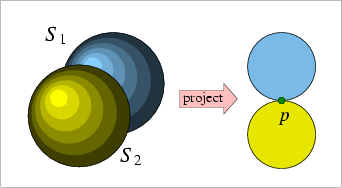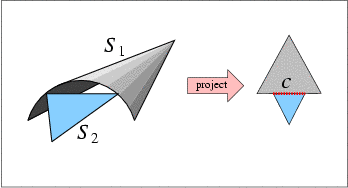(a) (b)
Figure 24.1:  (a) The spheres S1 and S2 have only one two-dimensional point p in their common xy-definition range. They do not necessarily intersect over this point, and the envelope-construction algorithm needs to determine their relative z-order over p. (b) The z-order of the surfaces S1 and S2 should be determined over the x-monotone curve c. The comparison is performed over the interior of c, excluding its endpoints.

• Subdivide a given surface into continuous xy-monotone surfaces. It is possible to disregard xy-monotone surfaces that do not contribute to the surface envelope at this stage (for example, if we are given a sphere, it is possible to return just its lower hemisphere if we are interested in the lower envelope; the upper hemisphere is obviously redundant).
• Given an xy-monotone surface S, construct all planar curves that form the boundary of the vertical projection S's boundary onto the xy-plane.

This operation is used at the bottom of the recursion to build the minimization diagram of a single xy-monotone surface.

• Construct all geometric entities that comprise the projection (onto the xy-plane) of the intersection between two xy-monotone surfaces S1 and S2. These entities may be:
• A planar curve, which is the projection of an 3D intersection curve of S1 and S2 (for example, the intersection curve between two spheres is a 3D circle, which becomes an ellipse when projected onto the xy-plane). In many cases it is also possible to indicate the multiplicity of the intersection: if it is odd, the two surfaces intersect transversely and change their relative z-positions on either side of the intersection curve; if it the multiplicity is even, they maintain their relative z-position. Providing the multiplicity information is optional. When provided, it is used by the algorithm to determine the relative order of S1 and S2 on one side of their intersection curve when their order on the other side of that curve is known, thus improving the performance of the algorithm.
• A point, induces by the projection of a tangency point of S1 and S2, or by the projection of a vertical intersection curve onto the xy-plane.
Needless to say, the set of intersection entities may be empty in case S1 and S2 do not intersect.
• Given two xy-monotone surfaces S1 and S2, and a planar point p = (x0,y0) that lies in their common xy-definition range, determine the z-order of S1 and S2 over p, namely compare S1(x0,y0) and S2(x0,y0). This operation is used only in degenerate situations, in order to determine the surface inducing the envelope over a vertex (see Figure 24.1(a) for an illustration of a situation when this operation is used).
• Given two xy-monotone surfaces S1 and S2, and a planar x-monotone curve c, which is a part of their projected intersection, determine the z-order of S1 and S2 immediately above (or, similarly, immediately below) the curve c. Note that c is a planar x-monotone curve, and we refer to the region above (or below) it in the plane. If c is a vertical curve, we regard the region to its left as lying above it, and the region to its right as lying below it.

This operation is used by the algorithm to determine the surface that induce the envelope over a face incident to c.

• Given two xy-monotone surfaces S1 and S2, and a planar x-monotone curve c, which fully lies in their common xy-definition range, and such that S1 and S2 do not intersect over the interior of c, determine the relative z-order of s1 and s2 over the interior of c. Namely, we compare S1(x0,y0) and S2(x0,y0) for some point (x0, y0) on c.

This operation is used by the algorithm to determine which surface induce the envelope over an edge associated with the x-monotone curve c, or of a face incident to c, in situations where the previous predicate cannot be used, as c is not an intersection curve of S1 and S2 (see Figure 24.1(b) for an illustration of a situation where this operation is used).

The package currently contains a traits class for named Env_triangle_traits_3<Kenrel> handling 3D triangles, and another named Env_sphere_traits_3<ConicTraits> for 3D spheres, based on geometric operations on conic curves (ellipses). In addition, the package includes a traits-class decorator that enables users to attach external (non-geometric) data to surfaces. The usage of the various traits classes is demonstrated in the next section.

## 24.3   Examples

### 24.3.1   Example for Envelope of Triangles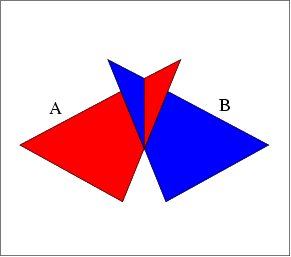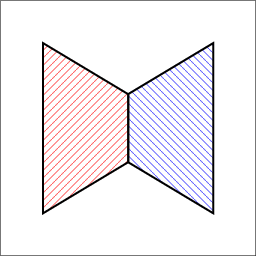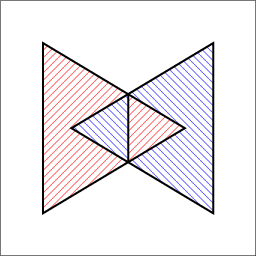(a) (b) (c)
Figure 24.2:  (a) Two triangles in3, as given in ex_envelope_triangles.cpp. (b) Their lower envelope. (c) Their upper envelope.

The following example shows how to use the envelope-traits class for 3D triangles and how to traverse the envelope diagram. It constructs the lower and upper envelopes of the two triangles, as depicted in Figure 24.2(a) and prints the triangles that induce each face and each edge in the output diagrams. For convenience, we use the traits-class decorator Env_surface_data_traits_3 to label the triangles. When printing the diagrams, we just output the labels of the triangles:

```File: examples/Envelope_3/ex_envelope_triangles.cpp
```
```#include <CGAL/Gmpq.h>
#include <CGAL/Cartesian.h>
#include <CGAL/Env_triangle_traits_3.h>
#include <CGAL/Env_surface_data_traits_3.h>
#include <CGAL/envelope_3.h>
#include <iostream>
#include <list>

typedef CGAL::Gmpq                                       Number_type;
typedef CGAL::Cartesian<Number_type>                     Kernel;
typedef CGAL::Env_triangle_traits_3<Kernel>              Traits_3;
typedef Kernel::Point_3                                  Point_3;
typedef Traits_3::Surface_3                              Triangle_3;
typedef CGAL::Env_surface_data_traits_3<Traits_3, char>  Data_traits_3;
typedef Data_traits_3::Surface_3                         Data_triangle_3;
typedef CGAL::Envelope_diagram_2<Data_traits_3>          Envelope_diagram_2;

/* Auxiliary function - print the features of the given envelope diagram. */
void print_diagram (const Envelope_diagram_2& diag)
{
// Go over all arrangement faces.
Envelope_diagram_2::Face_const_iterator            fit;
Envelope_diagram_2::Ccb_halfedge_const_circulator  ccb;
Envelope_diagram_2::Surface_const_iterator         sit;

for (fit = diag.faces_begin(); fit != diag.faces_end(); ++fit)
{
// Print the face boundary.
if (fit->is_unbounded())
{
std::cout << "[Unbounded face]";
}
else
{
// Print the vertices along the outer boundary of the face.
ccb = fit->outer_ccb();
std::cout << "[Face]  ";
do
{
std::cout << '(' << ccb->target()->point() << ")  ";
++ccb;
} while (ccb != fit->outer_ccb());
}

// Print the labels of the triangles that induce the envelope on this face.
std::cout << "-->  " << fit->number_of_surfaces()
<< " triangles:";

for (sit = fit->surfaces_begin(); sit != fit->surfaces_end(); ++sit)
std::cout << ' ' << sit->data();
std::cout << std::endl;
}

// Go over all arrangement edges.
Envelope_diagram_2::Edge_const_iterator            eit;

for (eit = diag.edges_begin(); eit != diag.edges_end(); ++eit)
{
// Print the labels of the triangles that induce the envelope on this edge.
std::cout << "[Edge]  (" << eit->source()->point()
<< ")  (" << eit->target()->point()
<< ")  -->  " << eit->number_of_surfaces()
<< " triangles:";

for (sit = eit->surfaces_begin(); sit != eit->surfaces_end(); ++sit)
std::cout << ' ' << sit->data();
std::cout << std::endl;
}

return;
}

/* The main program: */
int main ()
{
// Construct the input triangles, makred A and B.
std::list<Data_triangle_3>   triangles;

triangles.push_back (Data_triangle_3 (Triangle_3 (Point_3 (0, 0, 0),
Point_3 (0, 6, 0),
Point_3 (5, 3, 4)),
'A'));
triangles.push_back (Data_triangle_3 (Triangle_3 (Point_3 (6, 0, 0),
Point_3 (6, 6, 0),
Point_3 (1, 3, 4)),
'B'));

// Compute and print the minimization diagram.
Envelope_diagram_2      min_diag;

CGAL::lower_envelope_3 (triangles.begin(), triangles.end(),
min_diag);

std::cout << std::endl << "The minimization diagram:" << std::endl;
print_diagram (min_diag);

// Compute and print the maximization diagram.
Envelope_diagram_2      max_diag;

CGAL::upper_envelope_3 (triangles.begin(), triangles.end(),
max_diag);

std::cout << std::endl << "The maximization diagram:" << std::endl;
print_diagram (max_diag);

return (0);
}
```

### 24.3.2   Example for Envelope of Spheres

The next example demonstrates how to instantiate and use the envelope-traits class for spheres, based on the Arr_conic_traits_2 class that handles the projected intersecion curves. The program reads a set of spheres from an input file and constructs their lower envelope:

```File: examples/Envelope_3/ex_envelope_spheres.cpp
```
```#include <CGAL/basic.h>

#ifndef CGAL_USE_CORE
#include <iostream>
int main()
{
std::cout << "Sorry, this example needs CORE ..." << std::endl;
return 0;
}
#else

#include <CGAL/Cartesian.h>
#include <CGAL/CORE_algebraic_number_traits.h>
#include <CGAL/Arr_conic_traits_2.h>
#include <CGAL/Env_sphere_traits_3.h>
#include <CGAL/envelope_3.h>
#include <CGAL/Timer.h>
#include <iostream>
#include <list>

typedef CGAL::CORE_algebraic_number_traits            Nt_traits;
typedef Nt_traits::Rational                           Rational;
typedef Nt_traits::Algebraic                          Algebraic;
typedef CGAL::Cartesian<Rational>                     Rat_kernel;
typedef Rat_kernel::Point_3                           Rat_point_3;
typedef CGAL::Cartesian<Algebraic>                    Alg_kernel;

typedef CGAL::Arr_conic_traits_2<Rat_kernel, Alg_kernel, Nt_traits>
Conic_traits_2;

typedef CGAL::Env_sphere_traits_3<Conic_traits_2>     Traits_3;
typedef Traits_3::Surface_3                           Sphere_3;
typedef CGAL::Envelope_diagram_2<Traits_3>            Envelope_diagram_2;

int main(int argc, char **argv)
{
// Get the name of the input file from the command line, or use the default
// fan_grids.dat file if no command-line parameters are given.
const char * filename = (argc > 1) ? argv : "spheres.dat";

// Open the input file.
std::ifstream     in_file(filename);

if (! in_file.is_open())
{
std::cerr << "Failed to open " << filename << " ..." << std::endl;
return 1;
}

// Read the spheres from the file.
// The input file format should be (all coordinate values are integers):
// <n>                           // number of spheres.
// <x_1> <y_1> <x_1> <R_1>       // center and squared radious of sphere #1.
// <x_2> <y_2> <x_2> <R_2>       // center and squared radious of sphere #2.
//   :     :     :     :
// <x_n> <y_n> <x_n> <R_n>       // center and squared radious of sphere #n.
int                   n;
std::list<Sphere_3>   spheres;
int                   x, y, z, sqr_r;
int                   i;

in_file >> n;
for (i = 0; i < n; ++i)
{
in_file >> x >> y >> z >> sqr_r;
spheres.push_back(Sphere_3(Rat_point_3(x, y, z), Rational(sqr_r)));
}
in_file.close();

// Compute the lower envelope.
Envelope_diagram_2    min_diag;
CGAL::Timer           timer;

std::cout << "Constructing the lower envelope of "
<< n << " spheres." << std::endl;

timer.start();
CGAL::lower_envelope_3(spheres.begin(), spheres.end(), min_diag);
timer.stop();

// Print the dimensions of the minimization diagram.
std::cout << "V = " << min_diag.number_of_vertices()
<< ",  E = " << min_diag.number_of_edges()
<< ",  F = " << min_diag.number_of_faces() << std::endl;

std::cout << "Construction took " << timer.time()
<< " seconds." << std::endl;

return 0;
}

#endif
```

### 24.3.3   Example for Envelope of Planes

The next example demonstrates how to instantiate and use the envelope-traits class for planes, based on the Arr_linear_traits_2 class that handles infinite linear objects such as lines and rays.

```File: examples/Envelope_3/ex_envelope_planes.cpp
```
```#include <CGAL/Gmpq.h>
#include <CGAL/Cartesian.h>
#include <CGAL/Env_plane_traits_3.h>
#include <CGAL/envelope_3.h>
#include <iostream>
#include <list>

typedef CGAL::Gmpq                                       Number_type;
typedef CGAL::Cartesian<Number_type>                     Kernel;
typedef Kernel::Plane_3                                  Plane_3;
typedef CGAL::Env_plane_traits_3<Kernel>                 Traits_3;
typedef Traits_3::Surface_3                              Surface_3;
typedef CGAL::Envelope_diagram_2<Traits_3>               Envelope_diagram_2;

/* Auxiliary function - print the features of the given envelope diagram. */
void print_diagram (const Envelope_diagram_2& diag)
{
// Go over all arrangement faces.
Envelope_diagram_2::Face_const_iterator            fit;
Envelope_diagram_2::Ccb_halfedge_const_circulator  ccb;
Envelope_diagram_2::Surface_const_iterator         sit;

for (fit = diag.faces_begin(); fit != diag.faces_end(); ++fit)
{
// Print the face boundary.

// Print the vertices along the outer boundary of the face.
ccb = fit->outer_ccb();
std::cout << "[Face]  ";
do
{
if(!ccb->is_fictitious())
std::cout << '(' << ccb->curve() << ") ";
++ccb;
} while (ccb != fit->outer_ccb());

// Print the planes that induce the envelope on this face.
std::cout << "-->  " << fit->number_of_surfaces()
<< " planes:";

for (sit = fit->surfaces_begin(); sit != fit->surfaces_end(); ++sit)
std::cout << ' ' << sit->plane();
std::cout << std::endl;
}

return;
}

/* The main program: */
int main ()
{
// Construct the input planes.
std::list<Surface_3>   planes;

planes.push_back (Surface_3(Plane_3(0, -1, 1, 0)));
planes.push_back (Surface_3(Plane_3(-1, 0, 1, 0)));
planes.push_back (Surface_3(Plane_3(0, 1 , 1, 0)));
planes.push_back (Surface_3(Plane_3(1, 0, 1,  0)));

// Compute and print the minimization diagram.
Envelope_diagram_2      min_diag;

CGAL::lower_envelope_3 (planes.begin(), planes.end(), min_diag);

std::cout << std::endl << "The minimization diagram:" << std::endl;
print_diagram (min_diag);

// Compute and print the maximization diagram.
Envelope_diagram_2      max_diag;

CGAL::upper_envelope_3 (planes.begin(), planes.end(), max_diag);

std::cout << std::endl << "The maximization diagram:" << std::endl;
print_diagram (max_diag);

return (0);
}
```

### Footnotes

 1 We consider vertical surfaces, namely patches of planes that are perpendicular to the xy-plane, as weakly xy-monotone, to handle degenerate inputs properly.